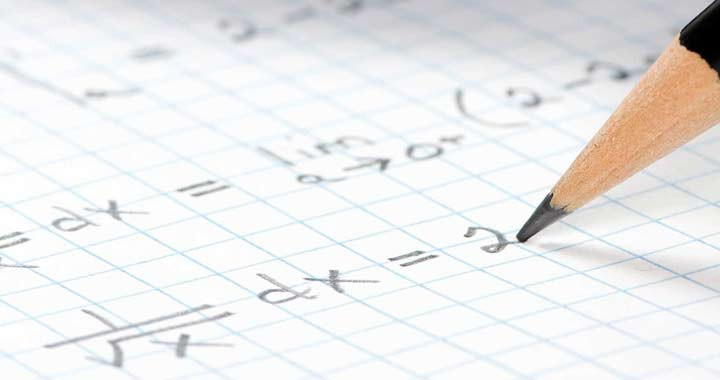# Mathematical SciencesMathematics is the international language of science and technology. Much of the subject matter in engineering and the natural sciences, as well as some social sciences such as economics, is presented in mathematical terms. Mathematical and statistical techniques are vital in fields that usually are not considered mathematical, such as biology, psychology, and political science.

Some students come to mathematical sciences with the intention of teaching in high school or college or pursuing research in mathematics. Some are attracted to mathematics for its own sake, for the beauty, discipline, logic, and problem-solving challenges. Other students pursue mathematics in order to achieve deeper understanding in their own areas of study.

Many students assume that most math majors become teachers. While many do, there are many other opportunities for math majors. The United States National Security Agency is the largest employer of math majors in the country. Math majors will also be found at NASA; in engineering firms; at insurance and risk management firms; in robotics and computer science companies; at large and small corporations working in market research, data management and web management; at social media start ups and established firms like YouTube; and any place that needs to make decisions based on numerical facts.

At UWM, the Math major has been designed to be flexible so that students could complete their major requirements via courses that match their interests and goals. Many students should find it relatively easy to complete double majors in mathematics and another subject.

Most mathematics courses belong in one of the following four groups: applied mathematics, computational mathematics, pure mathematics, and statistics.

• Applied Mathematics is a discipline using mathematical analysis to solve problems coming from outside the field of mathematics.
• Computational mathematics is closely related to applied mathematics. It emphasizes techniques of scientific computing and other computational analysis.
• Pure mathematics emphasizes the theory and structure underlying all areas of mathematics.
• Statistics is a field of mathematics that provides strategies and tools for using data to gain insight into real-world and experimental problems.

Students of the sciences, engineering, computer science, economics, and business often complete a significant number of mathematical sciences credits. These students are encouraged to take a mathematics major or minor, which adds an official recognition of important analytical skills valued by employers and graduate schools.

Students interested in teaching mathematics at the K-12 level should examine the School of Education programs.

Some students with an interest in computer science, choose to enroll in the Applied Math and Computer Science program. This program is ideal for the student who might have considered double majoring in both math and computer science; it combines applied math courses with computer science classes for you into one program.